# Generalized cohomology

• ## General Introduction

The phrase "generalized cohomology" is usually used to refer to a cohomology theory for topological spaces which satisfies the (generalized) Eilenberg-Steenrod axioms. The first such theory to appear, except for "ordinary" (singular) cohomology was topological K-theory.

<]]>

<]]>

<]]>
• ## Paper References

Kono and Tamaki: Generalized cohomology.

<]]>
• ## Definition

A generalized cohomology theory is a sequence of functors satisfying axioms I to VI below.

<]]>
• ## Properties

We present here the Eilenberg-Steenrod axioms, following Kono-Tamaki. Here we consider CW-complexes only, it would probably be better to consider compactly generated spaces.

We consider the category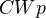of CW pairs, and the category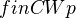of finite CW pairs. A CW pair is a pair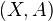consisting of a CW complex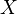and a subcomplex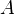, which can be empty.

There is a covariant endofunctor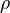onsendingto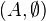.

We consider a sequence of contravariant functors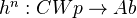together with natural transformations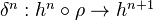for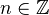.

Axioms:

• I, II, III: Functoriality of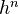and naturality of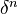• IV (Exactness): For any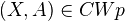, the following sequence is exact: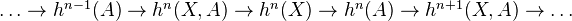• V (Homotopy): Homotopic maps ingive same map on cohomology

• VI (Excision): Let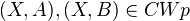. The inclusion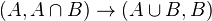induces an isomorphism on each cohomology group.
• VII (Dimension):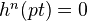for all nonzero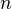.
• VIII (Additivity): "The cohomology of a disjoint union of spaces is the product of the cohomology of each space"
<]]>
• ## Standard theorems

Any cohomology theory satisfying axioms I - VI above has the following three exact sequences.

We say that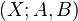is a triple ifand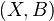are CW pairs. We say that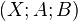is a triad ifand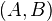are CW pairs.

Exact sequence for triple: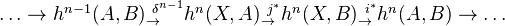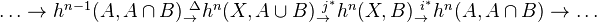where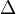is the excision isomorphism followed by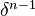.

Mayer-Vietoris exact sequence for a triad: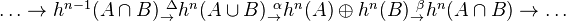where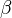is the difference map, and where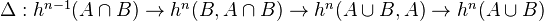, where the middle map is excision.

<]]>

<]]>

<]]>

<]]>

<]]>

<]]>

<]]>